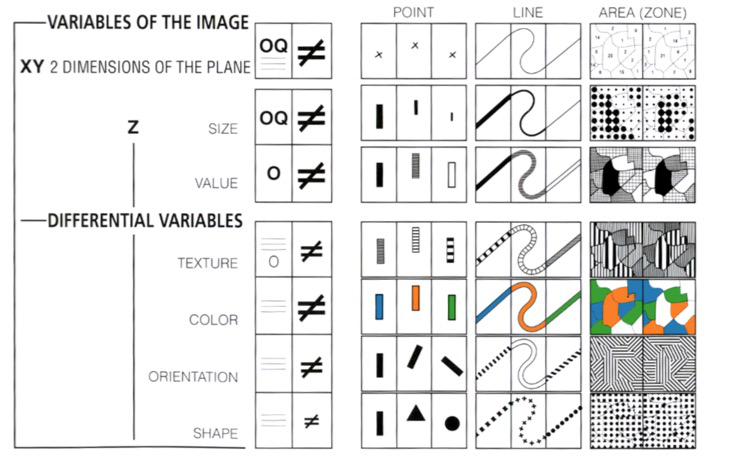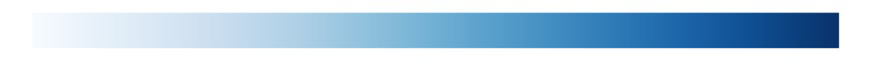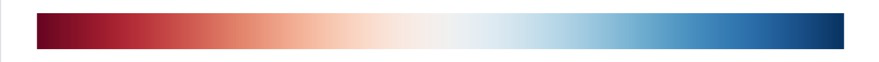# Continuous Scales

• linear, power, log, identity, time, or sequential color
• Following `continuous` stands for the returned object from one of the functions like `d3.scaleLinear()`

## `continuous(value)`reference

``````var x = d3.scaleLinear()
.domain([10, 130])
.range([0, 960]);

x(20); // 80
x(50); // 320``````
``````var color = d3.scaleLinear()
.domain([10, 100])
.range(["brown", "steelblue"]);

color(20); // "#9a3439"
color(50); // "#7b5167"``````

## `continuous.invert(value)`reference

• Inversion is useful for interaction, say to determine the data value corresponding to the position of the mouse.
• `continuous(continuous.invert(y))` approximately equals `y`
``````var x = d3.scaleLinear()
.domain([10, 130])
.range([0, 960]);

x.invert(80); // 20
x.invert(320); // 50``````

## `continuous.domain([domain])`reference

• If `domain` is specified, sets the scale’s domain to the specified array of numbers.
• If the elements in the given array are not numbers, they will be coerced to numbers.
``````var color = d3.scaleLinear()
.domain([-1, 0, 1])
.range(["red", "white", "green"]);

color(-0.5); // "rgb(255, 128, 128)"
color(+0.5); // "rgb(128, 192, 128)"``````

## `continuous.range([range])`reference

• Unlike the `domain`, elements in the given array need not be numbers
• Any value that is supported by the underlying `interpolator` will work

## `continuous.rangeRound([range])`reference

• Same as `.range()`, but use `d3.interpolateRound` instead of the default interpolator, which will round the resulting value to the nearest integer.
Equivalent to:
``````continuous
.range(range)
.interpolate(d3.interpolateRound);``````

## `continuous.clamp(clamp)`reference

• If `clamp` is specified, enables or disables clamping accordingly.
• If clamping is disabled and the scale is passed a value outside the domain, the scale may return a value outside the range through extrapolation.
``````var x = d3.scaleLinear()
.domain([10, 130])
.range([0, 960]);

x(-10); // -160, outside range
x.invert(-160); // -10, outside domain

x.clamp(true);
x(-10); // 0, clamped to range
x.invert(-160); // 10, clamped to domain``````

## `continuous.interpolate(interpolate)`reference

• The default interpolator may reuse return values.
• If the range values are objects, then the value interpolator always returns the same object, modifying it in-place.
A common reason to specify a custom interpolator is to change the color space of interpolation. For example, to use HCL:
``````var color = d3.scaleLinear()
.domain([10, 100])
.range(["brown", "steelblue"])
.interpolate(d3.interpolateHcl);``````

## `continuous.ticks([count])`reference

• Returns approximately `count` representative values from the scale’s domain.

## `continuous.tickFormat([count[, specifier]])`reference

``````var x = d3.scaleLinear()
.domain([-1, 1])
.range([0, 960]);

var ticks = x.ticks(5),
tickFormat = x.tickFormat(5, "+%");

ticks.map(tickFormat); // ["-100%", "-50%", "+0%", "+50%", "+100%"]``````

## `continuous.nice([count])`reference

• Extends the `domain` so that it starts and ends on nice round values.
• This method typically modifies the scale’s domain, and may only extend the bounds to the nearest round value.

# Introducing d3-scale tutorialSemiology of Graphics

A scale is a function that takes an abstract value of data, such as the mass of a diamond in carats, and returns a visual value such as the horizontal position of a dot in pixels. With two scales (one each for x and y), we have the basis for a scatterplot.

``````var x = d3.scaleLinear()
.domain(d3.extent(data, function(d) { return d.carat; }))
.range([0, width]);
var y = d3.scaleLinear()
.domain(d3.extent(data, function(d) { return d.price; }))
.range([height, 0]);``````

# Sequential Scales vs Diverging Scales discussion

• Both are similar to continuous scales
• However, unlike continuous scales, the output range is fixed by its interpolator and not configurable.

Examples of these types are as follows:

SequentialDiverging

Like `d3.scaleSequential`, but requires that the domain has three values, and maps to a diverging interpolator such as `d3.interpolateRdBu`.You can use these scales with interpolators provided by `d3-scale-chromatic`:

``let scale = d3.scaleSequential(d3.interpolateRdYlGn)``

# `d3-scale-chromatic`reference

``````var accent = d3.scaleOrdinal(d3.schemeAccent);
var blues = d3.scaleOrdinal(d3.schemeBlues);
var piyg = d3.scaleSequential(d3.interpolatePiYG);``````